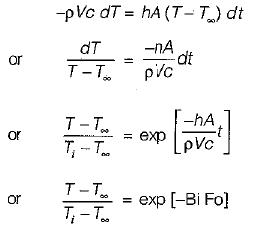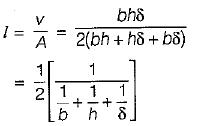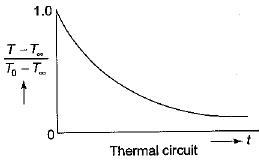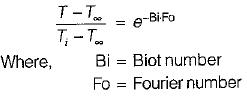Courses

# Transient Heat Conduction - 1

## 10 Questions MCQ Test Topicwise Question Bank for GATE Mechanical Engineering | Transient Heat Conduction - 1

Description
This mock test of Transient Heat Conduction - 1 for Mechanical Engineering helps you for every Mechanical Engineering entrance exam. This contains 10 Multiple Choice Questions for Mechanical Engineering Transient Heat Conduction - 1 (mcq) to study with solutions a complete question bank. The solved questions answers in this Transient Heat Conduction - 1 quiz give you a good mix of easy questions and tough questions. Mechanical Engineering students definitely take this Transient Heat Conduction - 1 exercise for a better result in the exam. You can find other Transient Heat Conduction - 1 extra questions, long questions & short questions for Mechanical Engineering on EduRev as well by searching above.
QUESTION: 1

Solution:
QUESTION: 2

### Which of the following statements is not correct? In a transient flow process

Solution:

Assumption: Change in internal energy of the billet during time

dt = Net heat flow from the billet to the bath or air during time dtQUESTION: 3

### Lumped parameter analysis for transient heat conduction is essentially valid for

Solution:
QUESTION: 4

Which of the following changes in temperature during unsteady state follow a periodic variation?

Solution:
QUESTION: 5

Lumped parameter analysis of transient heat conduction in solid stipulates
1. infinite thermal conductivity.
2. negligible temperature gradient i.e., practically uniform temperature within the solid.
3. small conduction resistance.
4. predominance of convective resistance

Which of the above statements are valid ?

Solution:
QUESTION: 6

Which of the followings is wrong value of characteristic length l which appears in the Biot number hl/k cand the Fourier number αζ / l2 ?

Solution:b and h >> δ
∴ l = δ/2

QUESTION: 7

The temperature distribution during transient heat conduction in a solid does not depend upon

Solution:
QUESTION: 8

The curve for unsteady state cooling or heating of bodies is

Solution:QUESTION: 9

In unsteady state heat conduction the two significant dimensionless parameter used are

Solution:

In case of unsteady state conductionQUESTION: 10

A copper block and an air mass block having similar dimensions are subjected to symmetrical heat transfer from one face of each block will reach to the same temperature at a rate

Solution:

Since thermal conductivity of copper is far greater than air.